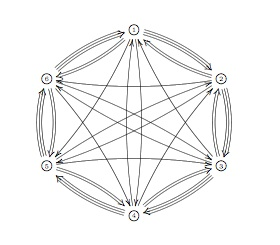# Tag: Kontsevich

After a lengthy spring-break, let us continue with our course on noncommutative geometry and $SL_2(\mathbb{Z})$-representations. Last time, we have explained Grothendiecks mantra that all algebraic curves defined over number fields are contained in the profinite compactification
$\widehat{SL_2(\mathbb{Z})} = \underset{\leftarrow}{lim}~SL_2(\mathbb{Z})/N$ of the modular group $SL_2(\mathbb{Z})$ and in the knowledge of a certain subgroup G of its group of outer automorphisms
. In particular we have seen that many curves defined over the algebraic numbers $\overline{\mathbb{Q}}$ correspond to permutation representations of $SL_2(\mathbb{Z})$. The profinite compactification $\widehat{SL_2}=\widehat{SL_2(\mathbb{Z})}$ is a continuous group, so it makes sense to consider its continuous n-dimensional representations $\mathbf{rep}_n^c~\widehat{SL_2}$ Such representations are known to have a finite image in $GL_n(\mathbb{C})$ and therefore we get an embedding $\mathbf{rep}_n^c~\widehat{SL_2} \hookrightarrow \mathbf{rep}_n^{ss}~SL_n(\mathbb{C})$ into all n-dimensional (semi-simple) representations of $SL_2(\mathbb{Z})$. We consider such semi-simple points as classical objects as they are determined by – curves defined over $\overline{Q}$ – representations of (sporadic) finite groups – modlart data of fusion rings in RCTF – etc… To get a feel for the distinction between these continuous representations of the cofinite completion and all representations, consider the case of $\hat{\mathbb{Z}} = \underset{\leftarrow}{lim}~\mathbb{Z}/n \mathbb{Z}$. Its one-dimensional continuous representations are determined by roots of unity, whereas all one-dimensional (necessarily simple) representations of $\mathbb{Z}=C_{\infty}$ are determined by all elements of $\mathbb{C}$. Hence, the image of $\mathbf{rep}_1^c~\hat{\mathbb{Z}} \hookrightarrow \mathbf{rep}_1~C_{\infty}$ is contained in the unit circle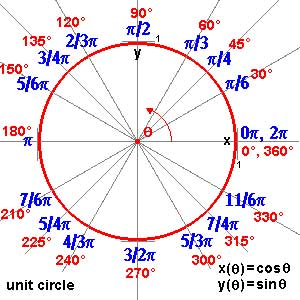and though these points are very special there are enough of them (technically, they form a Zariski dense subset of all representations). Our aim will be twofold : (1) when viewing a classical object as a representation of $SL_2(\mathbb{Z})$ we can define its modular content (which will be the noncommutative tangent space in this classical point to the noncommutative manifold of $SL_2(\mathbb{Z})$). In this way we will associate noncommutative gadgets to our classical object (such as orders in central simple algebras, infinite dimensional Lie algebras, noncommutative potentials etc. etc.) which give us new tools to study these objects. (2) conversely, as we control the tangentspaces in these special points, they will allow us to determine other $SL_2(\mathbb{Z})$-representations and as we vary over all classical objects, we hope to get ALL finite dimensional modular representations. I agree this may all sound rather vague, so let me give one example we will work out in full detail later on. Remember that one can reconstruct the sporadic simple Mathieu group $M_{24}$ from the dessin d’enfant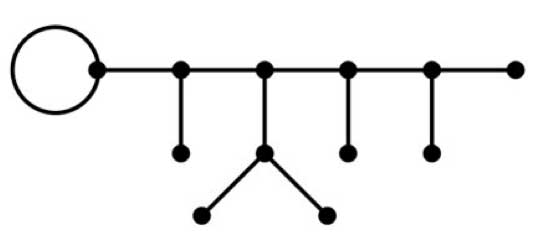This
dessin determines a 24-dimensional permutation representation (of
$M_{24}$ as well of $SL_2(\mathbb{Z})$) which
decomposes as the direct sum of the trivial representation and a simple
23-dimensional representation. We will see that the noncommutative
tangent space in a semi-simple representation of
$SL_2(\mathbb{Z})$ is determined by a quiver (that is, an
oriented graph) on as many vertices as there are non-isomorphic simple
components. In this special case we get the quiver on two points
$\xymatrix{\vtx{} \ar@/^2ex/[rr] & & \vtx{} \ar@/^2ex/[ll] \ar@{=>}@(ur,dr)^{96} }$ with just one arrow in each direction
between the vertices and 96 loops in the second vertex. To the
experienced tangent space-reader this picture (and in particular that
there is a unique cycle between the two vertices) tells the remarkable
fact that there is **a distinguished one-parameter family of
24-dimensional simple modular representations degenerating to the
permutation representation of the largest Mathieu-group**. Phrased
differently, there is a specific noncommutative modular Riemann surface
associated to $M_{24}$, which is a new object (at least as far
as I’m aware) associated to this most remarkable of sporadic groups.
Conversely, from the matrix-representation of the 24-dimensional
permutation representation of $M_{24}$ we obtain representants
of all of this one-parameter family of simple
$SL_2(\mathbb{Z})$-representations to which we can then perform
noncommutative flow-tricks to get a Zariski dense set of all
24-dimensional simples lying in the same component. (Btw. there are
also such noncommutative Riemann surfaces associated to the other
sporadic Mathieu groups, though not to the other sporadics…) So this
is what we will be doing in the upcoming posts (10) : explain what a
noncommutative tangent space is and what it has to do with quivers (11)
what is the noncommutative manifold of $SL_2(\mathbb{Z})$? and what is its connection with the Kontsevich-Soibelman coalgebra? (12)
is there a noncommutative compactification of $SL_2(\mathbb{Z})$? (and other arithmetical groups) (13) : how does one calculate the noncommutative curves associated to the Mathieu groups? (14) : whatever comes next… (if anything).

According to a science article in the New York Times, archeologists have discovered “signs of advanced math” in medieval mosaics. An example of a quasi-crystalline Penrose pattern was found at the Darb-i Imam shrine in Isfahan, Iran.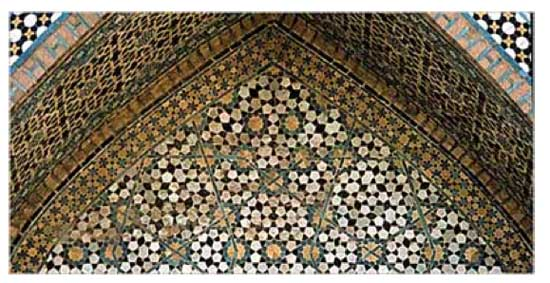“A new study shows that the Islamic pattern-making process, far more intricate than the laying of one‚Äôs bathroom floor, appears to have involved an advanced math of quasi crystals, which was not understood by modern scientists until three decades ago. Two years ago, Peter J. Lu, a doctoral student in physics at Harvard University, was transfixed by the geometric pattern on a wall in Uzbekistan. It reminded him of what mathematicians call quasi-crystalline designs. These were demonstrated in the early 1970s by Roger Penrose, a mathematician and cosmologist at the University of Oxford. Mr. Lu set about examining pictures of other tile mosaics from Afghanistan, Iran, Iraq and Turkey, working with Paul J. Steinhardt, a Princeton cosmologist who is an authority on quasi crystals and had been Mr. Lu’s undergraduate adviser. ”

Penrose tilings are one of the motivating examples of Alain Connes’ book as there is a $C^*$-algebra associated to it. In fact, the algebra is AF ( a limit of semi-simple finite dimensional algebras) so is even a formally smooth algebra in Kontsevichian noncommutative geometry (it is remarkable how quickly one gets used to silly terminology…). However, the Penrose algebra is simple, so rather useless from the point of view of finite dimensional representations… Still, Connesian noncommutative geometry may be a recent incarnation of the medieval Tehran program (pun intended). Thanks to easwaran for the link (via Technorati).

Added, March 1 : I haven’t looked at the Connes-Marcolli paper A walk in the noncommutative garden for a while but now that I do, I see that they mentioned the above already at the end of their section on Tilings (page 32). They also include clearer pictures.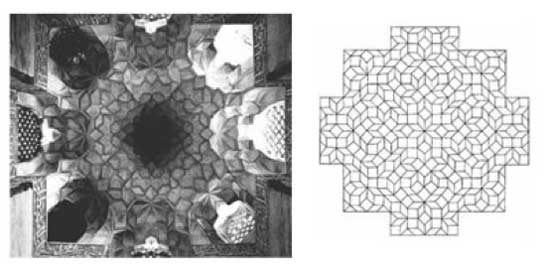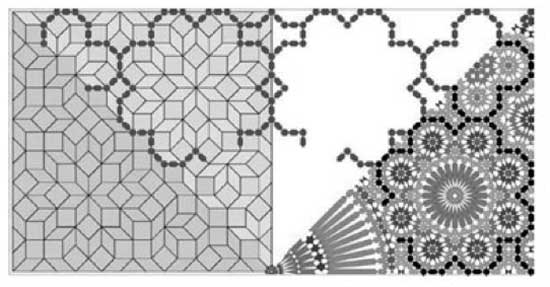At
last, some excitement about noncommutative geometry in the blogosphere.

From what I deduce from reading the first posts, Arup Pal set up a new blog
called Noncommutative
Geometry
and subsequently handed it over to Masoud Khalkhali who then
got Alain Connes to post on it who, in turn, is asking people to submit
posts, such as todays post by David Goss.

Somehow, most people refer to the Noncommutative Geometry blog as
“Alain Connes’ blog” (for example Doctor Gero, Not Even
Wrong
, the n-category cafe and
possibly many others).

David Corfield over at the n-category cafe stops
short of suggesting to rename (by analogy) NeverEndingBooks into
Kontsevich’s blog

A new blog Noncommutative Geometry
has begun, which appears to be of the Connesian variety. (Connes himself
has already commented there.) We mentioned a couple of weeks ago that
there are different flavours of noncommutative geometry. The
Kontsevichian variety, nongeometry, finds its blog voice in Lieven Le
Bruyn‚Äôs NeverEndingBooks. It would be interesting to see some
interaction.

I don’t think I will opt for a dialectic
response to the Noncommutative Geometry Blog, although I realize this would result
in more enjoyable reading for some of you…

For starters,
I’ve signed up to another flagship of noncommutative
_differential_ geometry : noncommutativegeometry.net, though it is unclear to me what action (if any) is
going on over there.

Further, I plan to move my talks at the master class
noncommutative geometry
to the virtual lecture room of this blog,
hoping to get the desired interaction. We’ll start later this week and
the pace will be pretty easy going. A tentative title might be
“Anabelian versus Noncommutative Geometry”.

Here are
my nominees for the 2006 paper of the year award in mathematics &
mathematical physics : in math.RA : math.RA/0606241
: Notes on A-infinity
algebras, A-infinity categories and non-commutative geometry. I
by

Maxim Kontsevich
and
Yan Soibelman
. Here is the abstract :

We develop
geometric approach to A-infinity algebras and A-infinity categories
based on the notion of formal scheme in the category of graded vector
spaces. Geometric approach clarifies several questions, e.g. the notion
of homological unit or A-infinity structure on A-infinity functors. We
discuss Hochschild complexes of A-infinity algebras from geometric point
of view. The paper contains homological versions of the notions of
properness and smoothness of projective varieties as well as the
non-commutative version of Hodge-to-de Rham degeneration conjecture. We
also discuss a generalization of Deligne’s conjecture which includes
both Hochschild chains and cochains. We conclude the paper with the
description of an action of the PROP of singular chains of the
topological PROP of 2-dimensional surfaces on the Hochschild chain
complex of an A-infinity algebra with the scalar product (this action is
more or less equivalent to the structure of 2-dimensional Topological
Field Theory associated with an “abstract” Calabi-Yau
manifold).

why ? : Because this paper
probably gives the correct geometric object associated to a
non-commutative algebra (a huge coalgebra) and consequently the right
definition of a map between noncommutative affine schemes. In a previous post (and its predecessors) I’ve
tried to explain how this links up with my own interpretation and since
then I’ve thought more about this, but that will have to wait for
another time. in hep-th : hep-th/0611082 : Children’s Drawings From
Seiberg-Witten Curves
by Sujay K. Ashok, Freddy Cachazo, Eleonora
Dell’Aquila. Here is the abstract :

We consider N=2
supersymmetric gauge theories perturbed by tree level superpotential
terms near isolated singular points in the Coulomb moduli space. We
identify the Seiberg-Witten curve at these points with polynomial
equations used to construct what Grothendieck called “dessins
d’enfants” or “children’s drawings” on the Riemann
sphere. From a mathematical point of view, the dessins are important
because the absolute Galois group Gal(\bar{Q}/Q) acts faithfully on
them. We argue that the relation between the dessins and Seiberg-Witten
theory is useful because gauge theory criteria used to distinguish
branches of N=1 vacua can lead to mathematical invariants that help to
distinguish dessins belonging to different Galois orbits. For instance,
we show that the confinement index defined in hep-th/0301006 is a Galois
invariant. We further make some conjectures on the relation between
Grothendieck’s programme of classifying dessins into Galois orbits and
the physics problem of classifying phases of N=1 gauge theories.

why ? : Because this paper gives the
best introduction I’ve seen to Grothendieck’s dessins d’enfants
(slightly overdoing it by giving a crash course on elementary Galois
theory in appendix A) and kept me thinking about dessins and their
Galois invariants ever since (again, I’ll come back to this later).

Last
time we saw that the _coalgebra of distributions_ of a
noncommutative manifold can be described as a coalgebra
Takeuchi-equivalent to the path coalgebra of a huge quiver. This
infinite quiver has as its vertices the isomorphism classes of finite
dimensional simple representations of the qurve A (the coordinate ring
of the noncommutative manifold) and there are as many directed arrows
between the vertices corresponding to the simples S and T as is the
dimension of $Ext^1_A(S,T)$.

The fact that this
coalgebra of distributions is equivalent to the path coalgebra of
_some_ quiver is in the Kontsevich-Soibelman
paper
though it would have been nice if they had given reference for
this fact to the paper Wedge Products and
Cotensor Coalgebras in Monoidal Categories
by Ardizzoni or to
previous work by P. Jara, D. Llena, L. Merino and D. Stefan,
“Hereditary and formally smooth coalgebras”, Algebr.
Represent. Theory 8 (2005), 363-374. In those papers it is shown that a
coalgebra with coseparable coradical is hereditary if and only if it
is formally smooth if and only if it is a cotensor coalgebra of some
bicomodule.

At first this looks just like the dual version of
the classical result that a finite dimensional hereditary algebra is
Morita equivalent to the path algebra of a quiver (which is indeed what
the proof does) but again the condition that the coradical is
coseparable does not require the coradical to be finite dimensional…
In our case, the coradical is indeed coseparable being the direct sum
over all matrix coalgebras corresponding to the simple representations.
Hence, we can again recover the _points_ of our noncommutative manifold
from the direct summands of the coradical. Fortunately, one can
compute this huge coalgebra of distributions from a small quiver, the
_one quiver to rule them all_, but as I’ve been babbling about all of
this here [numerous
times](http://www.neverendingbooks.org/?s=one+quiver) I’ll let the
interested find out for themselves how you use it (a) to get at the
isoclasses of all simples (hint : morally they are the smooth points of
the quotient varieties of n-dimensional representations and enough tools
have been developed recently to spot some fake simples, that is smooth
proper semi-simple points) and (b) to compute the _ragball_, that is the
huge quiver with vertex set the simples and arows as described
above. Over the years I’ve calculated several one-quivers for a
variety of qurves (such as amalgamated free products of finite groups
and smooth curves). If you are in for a puzzle, try to determine it for
the qurve $~(\mathbb{C}[x] \ast C_2) \ast_{\mathbb{C} C_2} \mathbb{C} PSL_2(\mathbb{Z}) \ast_{\mathbb{C} C_3} (\mathbb{C}[x] \ast C_3)$ The answer is a mysterious
hexagon